Courses

# Chemical Bonding & Periodicity

## 20 Questions MCQ Test GATE Chemistry Mock Test Series 2020 | Chemical Bonding & Periodicity

Description
This mock test of Chemical Bonding & Periodicity for Chemistry helps you for every Chemistry entrance exam. This contains 20 Multiple Choice Questions for Chemistry Chemical Bonding & Periodicity (mcq) to study with solutions a complete question bank. The solved questions answers in this Chemical Bonding & Periodicity quiz give you a good mix of easy questions and tough questions. Chemistry students definitely take this Chemical Bonding & Periodicity exercise for a better result in the exam. You can find other Chemical Bonding & Periodicity extra questions, long questions & short questions for Chemistry on EduRev as well by searching above.
*Answer can only contain numeric values
QUESTION: 1

### The number of species having 3c-2e- bond among following is/are____ Al2(CH3)6, AI2(CH3)4CI2,(MgH2)2, AI(BH4)3, BeCI2(in solid)

Solution:

Species in which ligands are electron deficient (one electron) form 3c–2e– bonding.

*Answer can only contain numeric values
QUESTION: 2

### The percentage s-character in lone pair in PH3 molecule having bond angle 92º is _____________

Solution: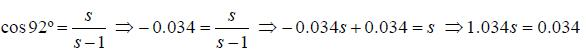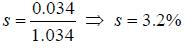Hence, s-character in lone pair 100-9.6 = 90.4%

QUESTION: 3

### Correct order of ionization energy among following is/are  (I) N2 > N > O    (II) N > O > O2    (III) N2 > O2 > NO    (IV) N2 > C2 > O2

Solution:

Energy required for removing electron from orbitals follows the order ABMO < A.O. < B.M.O.

QUESTION: 4

Species having highest vp-o value is

Solution:

As the electronegativity of substituted vP-O also increases

QUESTION: 5

Correct order of bond angle from following?

Solution:

Energy required for removing electron from orbitals follows the order ABMO < A.O. <B.M.O.

QUESTION: 6

The HOMO in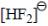molecule

Solution:

Molecular orbital diagram of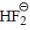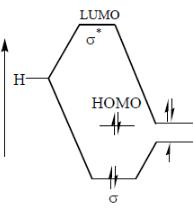QUESTION: 7

Correct set of point group for BrF5,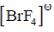and [BrF6]+ respectively are-

Solution: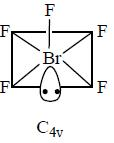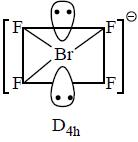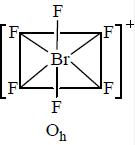QUESTION: 8

The correct statement is

Solution:

Lesser the electronegativity of surrounding atom more s-character in that bond. Hence, more bond angle.

*Answer can only contain numeric values
QUESTION: 9

The maximum number of atoms that may lie in the same plane in molecule P(CH3)3(CF3)2 is _______

Solution: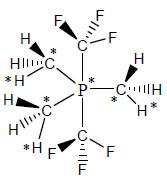*Answer can only contain numeric values
QUESTION: 10

Number of molecule that show fluxional behavior among. CH4,CF4,PCI5,PF5,BrF5,SF6,XeO3 is _________

Solution:
*Answer can only contain numeric values
QUESTION: 11

The number of anions having perfect Oh geometry among
[SbCI6]3-, [SeCI6]2-,[TeBr6]2-,[BrF6]-,[IF6]-,[CIF6]-,[SeF6]2- is/are__________

Solution:

Lesser the difference between size of central and surrounding atom lesser will be stereochemical activity of lone pair. Hence, perfect geometry.

QUESTION: 12

HOMO and LUMO in BeH2 molecules respectively are

Solution:

Molecular orbital diagram of BeH2.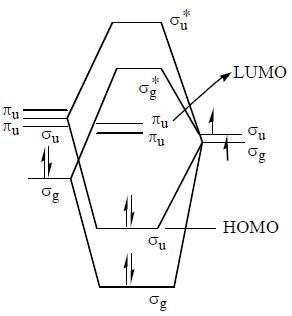QUESTION: 13

Regarding following compounds A,B,C and D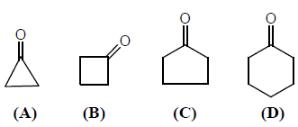Correct statement(s) is/are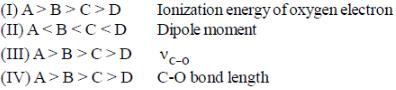Solution:

More the S-character in C-O bond = more will be strength of bond. Hence, more will be vc-o value. Hence, more ionization energy and lesser the dipole moment.

QUESTION: 14

The ionisation potential of hydrogen atom is 13.6 eV. The first ionisation potential of a sodium atom, assuming that the energy of its outer electron can be represented by a H-atom like model with an effective nuclear charge of 1.84, is

Solution: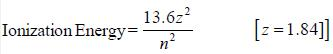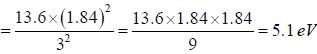QUESTION: 15

In salt [Et4N]2[InCI5] and [NH3(CH2)5(NH3)][TiCI5], structures of anions respectively are

Solution:

These structures are due to crystal packing effect.

QUESTION: 16

Select the correct  statement regarding [Ph3MeP][AI(BH4)4]
(I) At 298 anion show one signal in 1H NMR
(II) At 298 anion show two signal in 1H NMR
(III) At 298 a quintet is observed in 11B NMR
(IV) At 298 a triplet of triplet is observed in 11B NMR

Solution:

Structure of [AI(BH4)4]-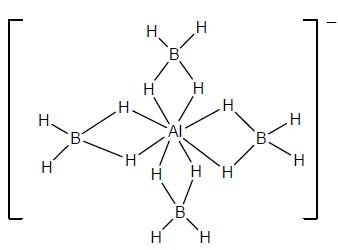At 298 terminal and bridging hydrogens are involved in dynamic process. Hence, one signal in 1H NMR. 11B NMR will be a quintet regarding 4 equivalent H.

QUESTION: 17

The ground state terms for H+2 and No respectively.

Solution: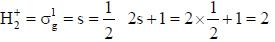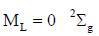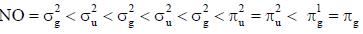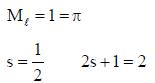Hence, 2πg

QUESTION: 18

29Si NMR spectrum of X[Ph3SiH2] shows

Solution:

Structure of X[Ph3,SiH2]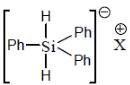Hence, triplet

QUESTION: 19

Geometry and point group regarding [ZrMe6]2-

Solution: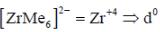perfect trigonal prismatic geometry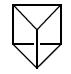perfect trigonal prismatic geometry has D3h point group.

*Answer can only contain numeric values
QUESTION: 20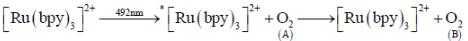The final dioxygen (B) undergo Diels Alder reaction unlike normal dioxygen(A). The value of spin multiplicity which  represent ground state term of dioxygen (B) is __________

Solution: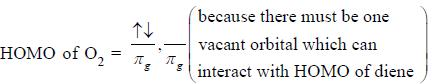2s + 1 = 1, Ml =2
Hence 1Δg